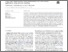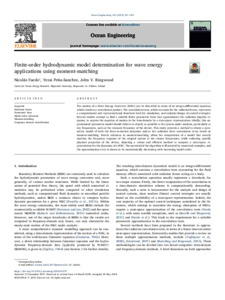# Finite-order hydrodynamic model determination for wave energy applications using moment-matching

Faedo, Nicolás and Peña-Sanchez, Yerai and Ringwood, John (2018) Finite-order hydrodynamic model determination for wave energy applications using moment-matching. Ocean Engineering, 163. pp. 251-263. ISSN 0029-8018Previewmore...Add this article to your Mendeley library

## Abstract

The motion of a Wave Energy Converter (WEC) can be described in terms of an integro-differential equation, which involves a convolution product. The convolution term, which accounts for the radiation forces, represents a computational and representational drawback both for simulation, and analysis/design of control strategies. Several studies attempt to find a suitable finite parametric form that approximates the radiation impulse response, to express the equation of motion in the time-domain by a state-space representation. Ideally, this approximated parametric model should behave as closely as possible to the system under analysis, particularly at key frequencies, such as the resonant frequency of the device. This study presents a method to obtain a parametric model of both the force-to-motion dynamics and/or the radiation force convolution term, based on moment-matching. Recent advances in moment-matching, allow the computation of a model that exactly matches the frequency response of the original system at the chosen frequencies, while enforcing specific physical properties of the device, depicting a robust and efficient method to compute a state-space representation for the dynamics of a WEC. The potential of the algorithm is illustrated by numerical examples, and the approximation error is shown to be monotonically decreasing with increasing model order.

Item Type: Article Radiation forces; Parametric form; Model order reduction; Moment-matching; Frequency-domain identification; Faculty of Science and Engineering > Electronic EngineeringFaculty of Science and Engineering > Research Institutes > Centre for Ocean Energy Research 12547 https://doi.org/10.1016/j.oceaneng.2018.05.037 Professor John Ringwood 06 Mar 2020 14:27 Ocean Engineering Elsevier Yes Science Foundation Ireland (SFI)Item control page